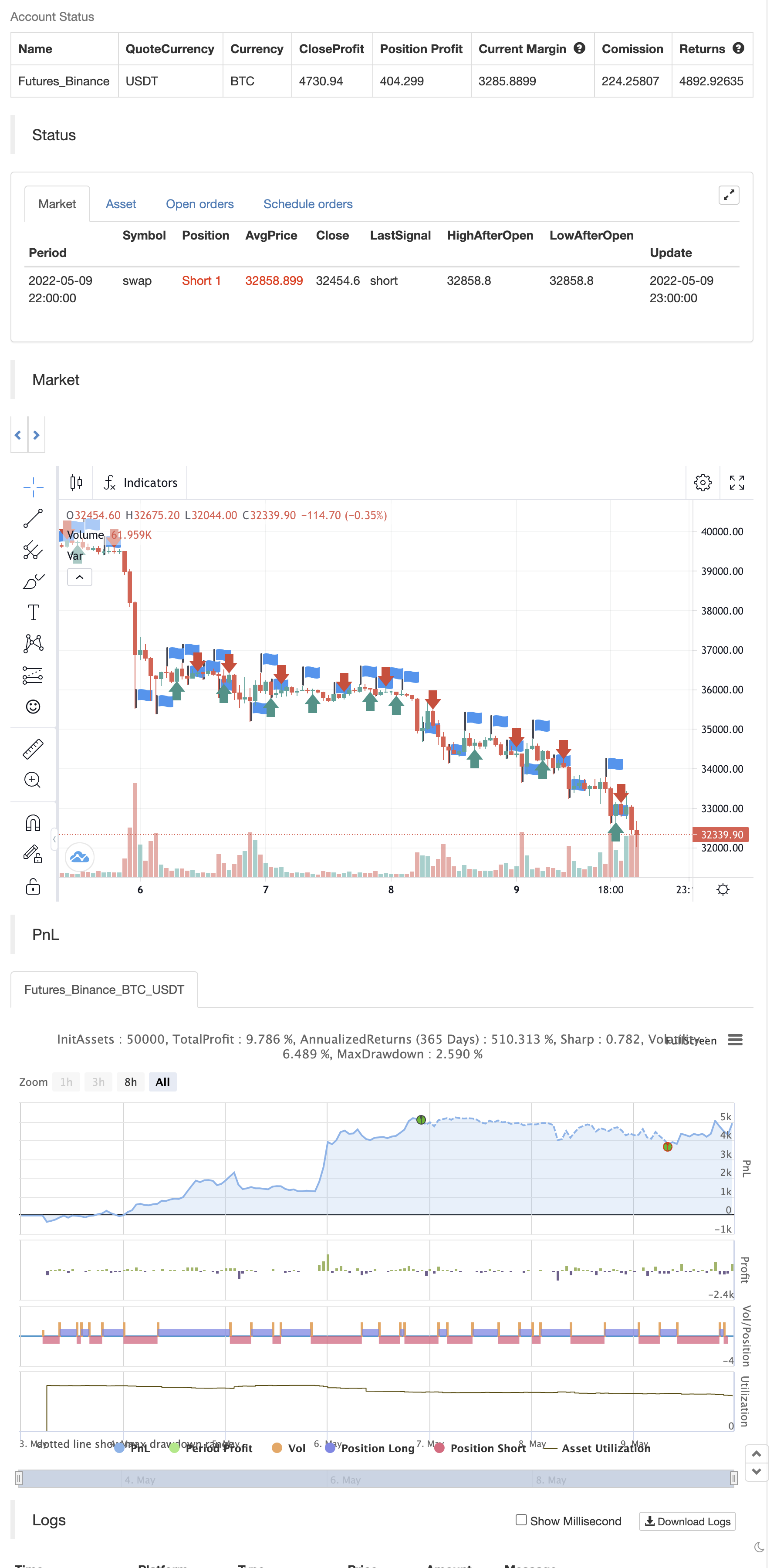# Smaller Fractals (+ Transparency)

Author: 张超, Date: 2022-05-11 14:05:06
Tags: Williams

Smaller Fractals (+ Transparency)

I find that fractals are super useful, but can visually clutter up the chart pretty quickly. Their opaqueness and just overall bigness can become a bit much.

As such, these are just like regular fractals, only smaller (pine script’s`size.tiny` instead of the default `size.small`).

Also, you can set a transparency level to these little guys (default is 50%).

Simple, more polite, and hopefully more useful fractals for those wnting a cleaner looking chart.

backtest```/*backtest
start: 2022-05-03 00:00:00
end: 2022-05-09 23:59:00
period: 1h
basePeriod: 15m
exchanges: [{"eid":"Futures_Binance","currency":"BTC_USDT"}]
*/

//@version=5
indicator("(Smaller) Williams Fractals", shorttitle="Tiny Fractals", format=format.price, precision=0, overlay=true)
// Define "n" as the number of periods and keep a minimum value of 2 for error handling.
n = input.int(title="Periods", defval=2, minval=2)

clr_trans = input.int(title="Fractal Transparency", defval=50)

// UpFractal
bool upflagDownFrontier = true
bool upflagUpFrontier0 = true
bool upflagUpFrontier1 = true
bool upflagUpFrontier2 = true
bool upflagUpFrontier3 = true
bool upflagUpFrontier4 = true

for i = 1 to n
upflagDownFrontier := upflagDownFrontier and (high[n-i] < high[n])
upflagUpFrontier0 := upflagUpFrontier0 and (high[n+i] < high[n])
upflagUpFrontier1 := upflagUpFrontier1 and (high[n+1] <= high[n] and high[n+i + 1] < high[n])
upflagUpFrontier2 := upflagUpFrontier2 and (high[n+1] <= high[n] and high[n+2] <= high[n] and high[n+i + 2] < high[n])
upflagUpFrontier3 := upflagUpFrontier3 and (high[n+1] <= high[n] and high[n+2] <= high[n] and high[n+3] <= high[n] and high[n+i + 3] < high[n])
upflagUpFrontier4 := upflagUpFrontier4 and (high[n+1] <= high[n] and high[n+2] <= high[n] and high[n+3] <= high[n] and high[n+4] <= high[n] and high[n+i + 4] < high[n])
flagUpFrontier = upflagUpFrontier0 or upflagUpFrontier1 or upflagUpFrontier2 or upflagUpFrontier3 or upflagUpFrontier4

upFractal = (upflagDownFrontier and flagUpFrontier)

// downFractal
bool downflagDownFrontier = true
bool downflagUpFrontier0 = true
bool downflagUpFrontier1 = true
bool downflagUpFrontier2 = true
bool downflagUpFrontier3 = true
bool downflagUpFrontier4 = true

for i = 1 to n
downflagDownFrontier := downflagDownFrontier and (low[n-i] > low[n])
downflagUpFrontier0 := downflagUpFrontier0 and (low[n+i] > low[n])
downflagUpFrontier1 := downflagUpFrontier1 and (low[n+1] >= low[n] and low[n+i + 1] > low[n])
downflagUpFrontier2 := downflagUpFrontier2 and (low[n+1] >= low[n] and low[n+2] >= low[n] and low[n+i + 2] > low[n])
downflagUpFrontier3 := downflagUpFrontier3 and (low[n+1] >= low[n] and low[n+2] >= low[n] and low[n+3] >= low[n] and low[n+i + 3] > low[n])
downflagUpFrontier4 := downflagUpFrontier4 and (low[n+1] >= low[n] and low[n+2] >= low[n] and low[n+3] >= low[n] and low[n+4] >= low[n] and low[n+i + 4] > low[n])
flagDownFrontier = downflagUpFrontier0 or downflagUpFrontier1 or downflagUpFrontier2 or downflagUpFrontier3 or downflagUpFrontier4

downFractal = (downflagDownFrontier and flagDownFrontier)

plotshape(downFractal, style=shape.triangledown, location=location.belowbar, offset=-n, color=color.new(#F44336,clr_trans), size = size.tiny)
plotshape(upFractal, style=shape.triangleup,   location=location.abovebar, offset=-n, color=color.new(#009688,clr_trans), size = size.tiny)

if upFractal
strategy.entry("Enter Long", strategy.long)
else if downFractal
strategy.entry("Enter Short", strategy.short)
```

Related

More